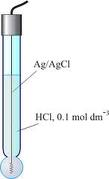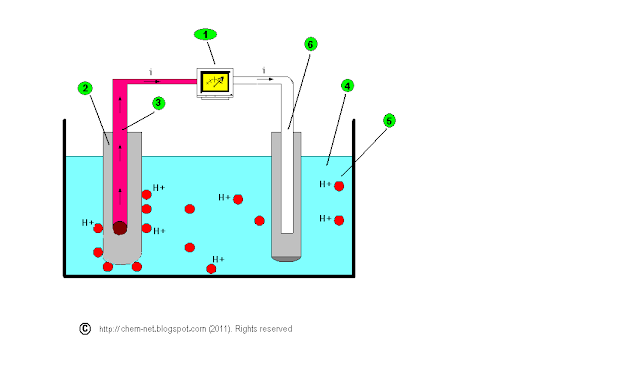Measuring the pH of a Solution with a pH meter | Chemistry Net

# Measuring the pH of a Solution with a pH meter

Measuring the pH of a Solution with a pH meter

# pH meter: Measuring the pH of a solution

The pH of a solution can be measured quickly and accurately with a pH meter (see Figure 1).

How does a pH meter work?

A pH meter has to somehow measure the concentration of the hydrogen ions [H+] in a solution. An acidic solution has far more positively charged hydrogen ions in it than an alkaline solution, so it has greater potential to produce an electric current under certain conditions - in other words, it is like a battery that can produce a greater voltage. A pH meter takes advantage of this and works like a typical voltameter: in brief, a pH meter consists of a pair of electrodes connected to a meter capable of measuring small voltages, on the order of millivolts. It measures the voltage (electrical potential) produced by the solution whose acidity we are interested in, compares it with the voltage of a known standard solution, and uses the difference in voltage (the potential difference) between them to calculate the difference in pH.What are the parts of a pH meter?

A typical pH meter consists of two parts: i) one special measuring probe (a glass electrode) or two measuring probes that are inserted into the solution whose pH is required and ii) an electronic meter that measures and displays the pH reading. A glass electrode is in a sense two electrodes combined in one. It consists of a long glass tube with a thin walled glass bulb at the end. Special glass of high electrical conductance and low melting point is used for the purpose. This glass can specifically sense hydrogen ions H+ up to a pH ≈ 9 (with special glass electrodes pH ranges from 1-13 can be measured). The bulb contains 0.1 M HCl and a Ag/AgCl electrode (used as an internal reference electrode) is immersed into the solution and connected by a platinum wire for electrical conduct.The main advantages of the glass electrode are:

• It can be used in the presence of strong oxidizing or reducing substances and metal ions
• Accurate results are obtained in the range pH 1-9. However, by using special glass electrodes pH 1-13 can be measured
• It is simple to operated. It can be attached to portable instruments and is used quite often in chemical, biological, industrial and agricultural laboratories

The main limitations of the glass electrode are:

• It does not function properly in some organic solvents (i.e. ethanol)
• It does not function properly above pH > 9 since it is sensitive to Na+ ions so a correction has to be made

In case that the pH meter has two probes (two electrodes): i) one of them is a glass electrode (has silver wire suspended in a solution of KCl that is contained in a special glass bulb coated with silica and metal salts) and ii) the other is the reference electrode and has a KCl wire suspended in a solution of KCl (see Figure 3).

• A higher voltage means more H+ ions in the solution and therefore a higher acidity. The pH meter shows in such a case a lower pH value since the solution is more acidic
• It does not function properly above pH > 9 since it is sensitive to Na+ ions so a correction has to be madeWhen the probe(s) are immersed into the solution some of the H+ ions in the solution move toward the glass electrode (Figure 3, labeled as 2) and replace some of the metal ions in its special surface. This creates a tiny current (voltage) that the silver electrode passes to the measuring device, the voltameter. The voltameter measures the voltage generated and shows a corresponding pH measurement as follows:

• A higher voltage means more H+ ions in the solution and therefore a higher acidity. The pH meter shows in such a case a lower pH value since the solution is more acidic
• It does not function properly above pH > 9 since it is sensitive to Na+ ions so a correction has to be made

The reference electrode (Figure 3, labeled as 6) acts as a reference for the measurement.

How accurate measurements can be made with a pH meter?

A calibrated instrument must be used for accurate measurements. A procedure for calibrating pH meters is given in the post Calibrating a pH meter

References

1. U.S. Pharmacopeia, 68, USP 36
2. David W. Oxtoby, H.P. Gillis, Alan Campion, “Principles of Modern Chemistry”, Sixth Edition, Thomson Brooks/Cole, 2008
3. Steven S. Zumdahl, “Chemical Principles” 6th Edition, Houghton Mifflin Company, 2009

Key Terms

pH meter, using a pH meter to measure pH, measuring the pH of a solution,